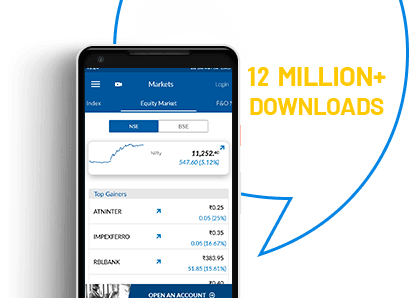# DIVIDEND YIELD CALCULATOR## Dividend Yield Calculator

One of the chief reasons for people buying shares is to earn a steady income in the form of dividends. Well-established companies pay dividends on a regular basis to their shareholders. Whenever firms make a profit, a part of it is distributed to the shareholders, who are part-owners of the company. Investors in dividend-paying stocks look for those with a high dividend yield. You can use a dividend yield calculator to find out if a stock is suitable for your needs or not.

DIVIDEND YIELD IS

It's important that the company pays out dividend on stocks for your long-term investment plan. It helps your investment to grow. There is a high demand for company stocks that pay a regular dividend. You must have also noticed that stocks prices make maximum movement around the time of dividend announcement. So, having a clear understanding of dividend will help you pick the right stocks.
Enjoy FREE Equity Delivery for Lifetime
Let's understand the dividend yield with the help of an example.

The dividend payment is variable. Companies announce dividend based on yearly performance. For ease of calculation, let's assume the company announces Rs 5 dividend for each share. Now, if the share price for the firm is Rs 100, then dividend yield value equals annualise dividend paid divided by price per share. In this example dividend yield is equal to 5 percent.

The process to calculate the dividend yield is complicated and time-intensive. This is why a dividend yield calculator is an absolute lifesaver. It is an automated computation tool that uses user input to calculate dividend payout – annual dividend per share, quarterly dividend, or total dividend, quickly and accurately.

Dividends create a passive stream of income for investors. Company stocks that have a good track record of paying high and stable dividend yield see consistently high demand for their shares among investors.

Angel One dividend yield calculator is a simple tool that anyone can use, and to use it, you wouldn't have to learn the complicated dividend yield formula. It also lets you compare high yield stocks to find the ones that suit your profile.

## How do you calculate dividend yield?

One can calculate dividend yield using a simple formula of dividing the total annual dividend paid per share by the price of individual equity.

Let's understand with an example. A company pays out Rs 5 dividend, and the price per share is Rs 150. Then dividend yield is 3.33 percent, calculated dividing dividend per share by its price.

Since it is challenging to calculate dividend yield manually, investors use an online dividend yield calculator that gives quick and accurate results.

## How do you calculate dividend payout?

Investors commonly calculate the dividend payout ratio to estimate how much a company pays to its investors as dividend and how much it retains for reinvesting and debt repayment. Simply saying, the dividend payout ratio (DPR) is the dividend paid against net income.

Investors use the following formula to calculate DPR.

DPR= DP/NI
DP = dividend paid
NI = net income

## What is a good dividend yield for a stock?

Dividend yield allows investors to measure the profit of each rupee they invest in and the potential risk of the particular investment. Though 'good' dividend yield value would depend on the industry, market condition, and price of shares, most investors consider a 2 to 6 percent dividend on stocks a good return.

Enjoy Zero Brokerage on Stock Investments